## A Model for Predicting Loan Default: Part II -- Model Building and Evaluation

LoanDefaultPart-II

# Loan Default Predictive Model: Model Building and Evaluation¶

In this blog post, I will be utilizing publicly available Lending Club loans' data to build a model to predict loan default. In the process, I will be demonstrating various techniques for data munging, data exploration, feature selection, model building based on several Machine Learning algorithms, and model evaluation to meet specific project goals.

LendingClub.com is a company that matches individual investors hoping to make good returns on an investment with borrowers looking for personal unsecured loans with reasonable fixed rates. It bills itself as "America's #1 credit marketplace" and is currently the largest peer-to-peer lending platform in the world. To request a loan, an individual completes an exhaustive application online. The application is then assessed for risk and awarded a grade ranging from A to G with G being the more risky ones. The grade awarded also determines the interest rate on the loan. Once approved, the loan is listed and investors can then browse loan listings and select loans to fund based on information on the borrower.

### Goal¶

For this project, I will be aiming to build a predictive model that will allow an investor to make well informed decisions on what loans to invest in so as to maximize on returns. Since the goal is to ensure that we avoid loan defaults, it is of paramount importance that I avoid misclassifying bad loans as good (a classification of a good loan as bad, while reducing the potential pool for investment, will not have the same ramifications).

### A Note on the Data¶

Data was obtained from the Lending Club website at https://www.lendingclub.com/info/download-data.action. For the analysis, I will be combining two sets of data, from 2007-2011 (9.64 Mb) and from 2012-2013 (37 Mb)

In :
import numpy as np
import pandas as pd
import sklearn
import matplotlib.pyplot as plt
%matplotlib inline
import seaborn.apionly as sns
import cPickle

pd.set_option('display.max_rows', 500)
pd.set_option('display.max_columns', 500)


It is important to check the version of scikitlearn because some features have changes, especially in version 0.18.

In :
sklearn.__version__

Out:
'0.18'

# Building a Predictive Model¶

Load the previously pickled data frame (see part I for this)

In :
df = pd.read_pickle('df_20072013_clean.pkl')


In :
corrmatrix = df.corr().abs()
corrmatrix = corrmatrix.stack()
corrmatrix[(corrmatrix > 0.6) & (corrmatrix != 1.0)].sort_values(ascending=True)

Out:
verification_status_Verified      verification_status_Not Verified    0.639063
verification_status_Not Verified  verification_status_Verified        0.639063
total_acc                         open_acc                            0.672061
open_acc                          total_acc                           0.672061
pub_rec                           pub_rec_bankruptcies                0.791670
pub_rec_bankruptcies              pub_rec                             0.791670
home_ownership_MORTGAGE           home_ownership_RENT                 0.849643
home_ownership_RENT               home_ownership_MORTGAGE             0.849643
funded_amnt_inv                   installment                         0.951947
installment                       funded_amnt_inv                     0.951947
loan_amnt                           0.956760
loan_amnt                         installment                         0.956760
installment                       funded_amnt                         0.962551
funded_amnt                       installment                         0.962551
funded_amnt_inv                   loan_amnt                           0.985186
loan_amnt                         funded_amnt_inv                     0.985186
funded_amnt_inv                   funded_amnt                         0.989663
funded_amnt                       funded_amnt_inv                     0.989663
loan_amnt                           0.996275
loan_amnt                         funded_amnt                         0.996275
dtype: float64
In :
highcorrelated_col = ['funded_amnt', 'installment', 'funded_amnt_inv']
df.drop(highcorrelated_col, axis=1, inplace=True)


Split the data to train and test

In :
from sklearn.model_selection import train_test_split #(new with scikit-learn 0.18)
#from sklearn.cross_validation import train_test_split (for pre scikit-learn 0.18)
X = df.iloc[:,1:]
y = df.iloc[:,0]
X_train, X_test, y_train, y_test = train_test_split(X, y, test_size=0.2, random_state=5)


The labels are y and the features X. Since sklearn.cross_validation.train_test_split does a random split every time it is called, specifying a random_state value ensures the split can be duplicated. The split ratio in this case is train:test = 80:20

### We are going to be building models over several algorithms: Logistic Regression, Random Forest, Naive Bayes, and Gradient Boosted Regression. In the first step, we will use a grid search with cross validation to optimize on the hyperparameters of each algorithm.¶

Set up an automatic workflow with pipeline

In :
from sklearn.preprocessing import StandardScaler
from sklearn.linear_model import LogisticRegression as LR
from sklearn.ensemble import RandomForestClassifier as RF
from sklearn.naive_bayes import GaussianNB as GNB
from sklearn.ensemble import GradientBoostingClassifier as GBC
from sklearn.decomposition import PCA
#from sklearn.pipeline import Pipeline
from imblearn.over_sampling import SMOTE
from imblearn.pipeline import Pipeline

/home/concinte/miniconda2/lib/python2.7/site-packages/sklearn/cross_validation.py:44: DeprecationWarning: This module was deprecated in version 0.18 in favor of the model_selection module into which all the refactored classes and functions are moved. Also note that the interface of the new CV iterators are different from that of this module. This module will be removed in 0.20.
"This module will be removed in 0.20.", DeprecationWarning)

In :
lr_pipeline = Pipeline([('smt', SMOTE(random_state=5, kind='borderline1', ratio ='auto')),
('scaler',StandardScaler()),
('classifier', LR(random_state = 5))
])

rf_pipeline = Pipeline([('smt', SMOTE(random_state=5, kind='borderline1', ratio ='auto')),
('scaler',StandardScaler()),
('classifier', RF(n_estimators=20, random_state = 5))
])

nb_pipeline = Pipeline([('smt', SMOTE(random_state=5)),
('scaler',StandardScaler()),
('classifier', GNB())
])

gb_pipeline = Pipeline([('smt', SMOTE(random_state=5)),
('scaler',StandardScaler()),
('classifier', GBC(random_state = 5))
])


The use of pipelines will ensure that feature extraction and modeling is restricted to the data in my training set, and there is no leakage of information to the test data. In its use, the feature extraction and modeling is restricted to each fold of a k-fold during cross-validation.

### Hyperparameter optimization¶

Use gridsearch and cross validation to optimize on the models hyperparameters

In :
#from sklearn.grid_search import GridSearchCV #pre version 0.18
from sklearn.model_selection import GridSearchCV #new with version 0.18

#Grid search for logistic regression
lr_param_range = [ 0.001, 0.01, 0.1]
lr_class_weight = [{0:0.01, 1:0.99}, {0:0.80, 1:0.20}]
lr_param_grid = [{'classifier__C':lr_param_range,
'classifier__class_weight':lr_class_weight}]
gridsearch_lr = GridSearchCV(estimator = lr_pipeline,
param_grid = lr_param_grid,
n_jobs = -1,
cv = 5)

#Grid search for random forest
rf_class_weight = [{0:0.01, 1:0.99}, {0:0.10, 1:0.90}, {0:0.80, 1:0.20}]
rf_param_grid =[{'classifier__max_features': ["sqrt"],
'classifier__class_weight':rf_class_weight,
'classifier__min_samples_split': [100, 200],
'classifier__min_samples_leaf': [5, 10],
'classifier__n_estimators': [20, 50],
'classifier__criterion': ["entropy"]}]
gridsearch_rf = GridSearchCV(estimator = rf_pipeline,
param_grid = rf_param_grid,
n_jobs = -1,
cv = 2)

#Grid search for Gradient Boosting Classifier
gb_param_grid = [{'classifier__n_estimators': ,
'classifier__min_samples_leaf': [9, 13],
'classifier__max_features': ['sqrt'],
'classifier__learning_rate': [0.05,  0.01],
}]
gridsearch_gb = GridSearchCV(estimator = gb_pipeline,
param_grid = gb_param_grid,
n_jobs = -1,
cv = 2)


Note: the parameters have to be assigned to the named step in the pipeline, in this case "classifier" by prepending "classifier" to the parameters with a double underscore, i.e., "classifier__C". See http://stackoverflow.com/questions/34889110/random-forest-with-gridsearchcv-error-on-param-grid. Note too that setting n_jobs (the number of distributed jobs) to -1 sets it to the number of cores. gridsearch_logreg is a GridSearch instance that behaves like a scikit-learn model.

Obtain the optimized hyperparameters for each algorithm by doing a fit to the data. Due to the size of the data set, I will initially only do grid search on a subest of the already randomized data

We need to know the total number of entries so that we can take half of them

In :
df.info(verbose=False)

<class 'pandas.core.frame.DataFrame'>
RangeIndex: 190040 entries, 0 to 190039
Columns: 41 entries, loan_status_binary to purpose_wedding
dtypes: float64(15), int64(2), uint8(24)
memory usage: 29.0 MB

In :
df_half = df[0:95000]


Extract the features and target that will be used for hyperparameter optimization.

In :
X_gs_train = df_half.iloc[:,1:]
y_gs_train = df_half.iloc[:,0]


Fit the data set to get the hyperparameters and pickle the best_estimator to be used later. I will use dill which is very robust at serialization to disk

In :
import dill

In [ ]:
gridsearch_lr.fit(X_gs_train, y_gs_train)
gridsearch_best_estimator_lr = gridsearch_lr.best_estimator_
dill.dump(gridsearch_best_estimator_lr, open('LogisticRegression_gridsearch_classweight.pkl', 'wb'))

gridsearch_rf.fit(X_gs_train, y_gs_train)
gridsearch_best_estimator_rf = gridsearch_rf.best_estimator_
dill.dump(gridsearch_best_estimator_rf, open('RandomForest_gridsearch_classweight.pkl', 'wb'))

gridsearch_gb.fit(X_gs_train, y_gs_train)
gridsearch_best_estimator_gb = gridsearch_gb.best_estimator_


### Model training and evaluation¶

With the optimized hyperparameters, we can then go ahead and fit models with cross validation. I will also be doing an evaluation of each model for accuracy, precision, recall, as well as the area under the Receiver Operating Characteristic (ROC) curve

This function will be used to draw the confusion matrix

In :
def draw_ConfusionMatrix(conf_matrix, classifier_name):
''' The confusion matrix draw function'''
fig, ax = plt.subplots(figsize=(4.5, 4.5))
ax.matshow(conf_matrix, cmap=plt.cm.Greens, alpha=0.3)
for i in range(conf_matrix.shape):
for j in range(conf_matrix.shape):
ax.text(x=j, y=i, s=conf_matrix[i, j], va='center', ha='center')

plt.title('Confusion Matrix for %s' % classifier_name)
plt.xlabel('Predicted label')
plt.ylabel('True label')

plt.tight_layout()
plt.show()


In the run_cv function, I will apply the cross-validation as well as draw the ROC curves for each training-validation fold.
Area Under Curve (AUC) plots are independent of the fraction of each class and are therefore a very good metric for evaluating a classifier performance in the case of when you are dealing with an imbalanced data set such as this one.

In :
from sklearn.cross_validation import KFold
from sklearn.metrics import accuracy_score
from sklearn.metrics import confusion_matrix
from sklearn.metrics import roc_curve, auc
from sklearn.metrics import classification_report
from scipy import interp

In :
def run_cv(X, y, classifier, clf_name):

#Construct a kfolds object
kf = KFold(len(y),n_folds=5,shuffle=True)

accuracy_scores = []

#Initialize ROC variables
mean_tpr = 0.0
mean_fpr = np.linspace(0, 1, 100)
all_tpr = []

clf = classifier

y_pred_full = y.copy()

#Iterate through folds
for i,(train_index, test_index) in enumerate(kf):

#Obtain the training and validation data sets for each fold
X_train, X_test = X.iloc[train_index], X.iloc[test_index]
y_train = y.iloc[train_index]
y_test = y.iloc[test_index]

#Train the classifier on the training data
clf_fit = clf.fit(X_train,y_train)

#Obtain a prediction on the test set
y_pred = clf_fit.predict(X_test)

#Map the prediction for this fold to the full dataset
y_pred_full.iloc[test_index] = y_pred

#Calculate the accuracy of the prediction on current fold
accuracy_scores.append(accuracy_score(y_true=y_test, y_pred=y_pred))

#Get probabilities and compute area under ROC curve
probas_ = clf_fit.predict_proba(X_test)
fpr, tpr, thresholds = roc_curve(y_test, probas_[:, 1])
mean_tpr += interp(mean_fpr, fpr, tpr)
mean_tpr = 0.0
roc_auc = auc(fpr, tpr)
plt.plot(fpr, tpr, lw=1, label='ROC fold %d (area = %0.2f)' % (i, roc_auc))

#Get Evaluation metrics
#Draw ROC Curve
mean_tpr /= len(kf)
mean_tpr[-1] = 1.0
mean_auc = auc(mean_fpr, mean_tpr)

plt.plot([0, 1],
[0, 1],
'--',
color=(0.6, 0.6, 0.6),
label='Luck')

plt.plot([0, 0, 1],
[0, 1, 1],
lw=2,
linestyle=':',
color='black',
label='Perfect Performance')

plt.plot(mean_fpr,
mean_tpr,
'k--',
label='Mean ROC (area = %0.2f)' % mean_auc, lw=2)

plt.xlim([-0.05, 1.05])
plt.ylim([-0.05, 1.05])
plt.xlabel('False Positive Rate')
plt.ylabel('True Positive Rate')
plt.title('Receiver Operating Characteristic for %s' % classifier_name)
plt.legend(loc="lower right")
#plt.tight_layout()
plt.show()

#Accuracy score
mean = np.mean(accuracy_scores)
std = np.std(accuracy_scores)
print clf_name + ':' + '\n' + 'cross-validation accuracy'
print "%.2f +/- %.3f" % (mean, std)
print classification_report(y, y_pred_full)

#Confusion Matrix
conf_matrix = confusion_matrix(y_true=y, y_pred=y_pred_full)
draw_ConfusionMatrix(conf_matrix, clf_name)

return clf_fit


Get the previously pickled classifiers with the optimized hyperparameters...

In :
import dill
with open('LogisticRegression_gridsearch_classweight.pkl', 'rb') as f:
with open('RandomForest_gridsearch_classweight.pkl', 'rb') as f:


...and run cross-validation on each algorithm for comparison

In :
classifier_name = 'Logistic Regression Classifier'
model_pipeline_lr = run_cv(X_train, y_train, LogisticRegression_classifier, classifier_name)

classifier_name = 'Random Forest Classifier'
model_pipeline_rf = run_cv(X_train, y_train, RandomForest_classifier, classifier_name)
dill.dump(model_pipeline_rf, open('RandomForest_model_AllFeatures.pkl', 'wb'))

classifier_name = 'Gaussian Naive Bayes'
GaussianNB_classifier = GNB()
model_pipeline_nb = run_cv(X_train, y_train, GaussianNB_classifier, classifier_name)

model_pipeline_gb = run_cv(X_train, y_train, GradientBoosting_classifier, classifier_name)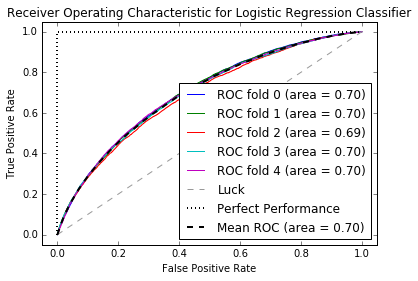Logistic Regression Classifier:
cross-validation accuracy
0.18 +/- 0.002
precision    recall  f1-score   support

0       0.96      0.01      0.02    126148
1       0.17      1.00      0.29     25884

avg / total       0.83      0.18      0.07    152032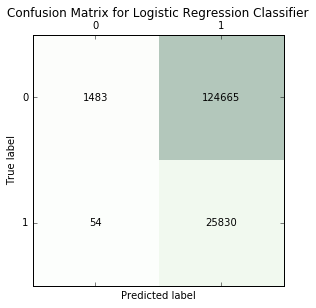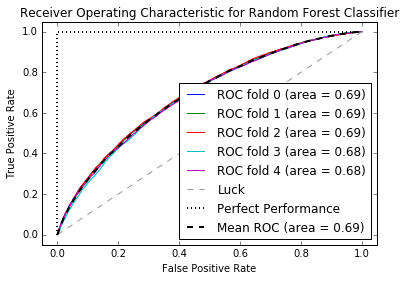Random Forest Classifier:
cross-validation accuracy
0.47 +/- 0.004
precision    recall  f1-score   support

0       0.92      0.40      0.56    126148
1       0.22      0.83      0.35     25884

avg / total       0.80      0.47      0.52    152032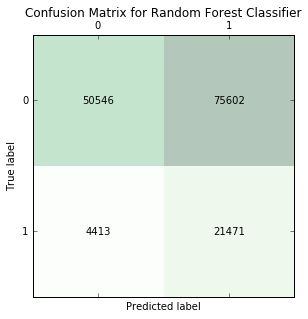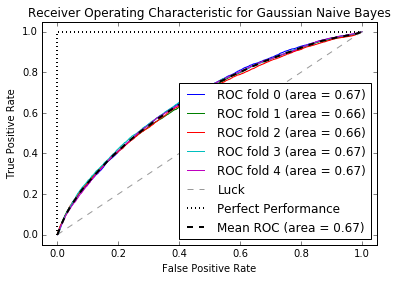Gaussian Naive Bayes:
cross-validation accuracy
0.82 +/- 0.006
precision    recall  f1-score   support

0       0.84      0.97      0.90    126148
1       0.41      0.11      0.17     25884

avg / total       0.77      0.82      0.78    152032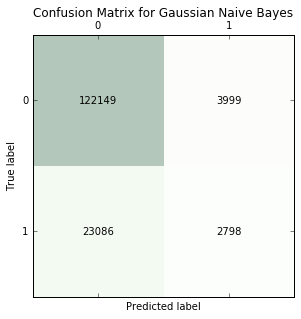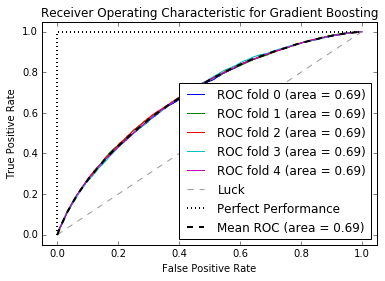Gradient Boosting:
cross-validation accuracy
0.82 +/- 0.001
precision    recall  f1-score   support

0       0.84      0.97      0.90    126148
1       0.41      0.10      0.16     25884

avg / total       0.77      0.82      0.78    152032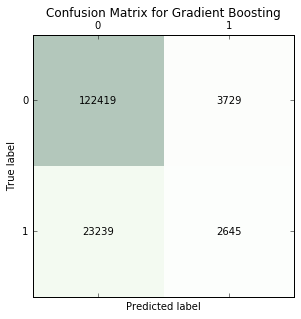### Ranking of Features¶

We will obtain a ranking of each feature using the trained Random Forest Classifier

Extract the classifier and trained forest from pipeline

In :
with open('RandomForest_model_AllFeatures.pkl', 'rb') as f:

In :
classifier = model_pipeline_rf.steps[-1]
forest = classifier


These are the feature importances. It consists of an array of each feature's importance score

In :
importances = forest.feature_importances_


Calculate the standard deviation in the feature importance for each tree of the random forest

In :
std = np.std([tree.feature_importances_ for tree in forest.estimators_],
axis=0)


Obtain the indices that will sort the importances array starting with the most important feature

In :
indices = np.argsort(importances)[::-1]


Get a list of the features

In :
features = X_train.columns


Rank each feature's importance and plot the top 10 most important features for easier visualization

In :
for f in range(X_train.shape):
print f+1, features[indices[f]], importances[indices[f]]

plt.figure()
plt.title("Feature importances")
plt.bar(range(10), importances[indices[:10]], yerr=std[indices[:10]], color="r", align="center")
plt.xticks(rotation=90)
plt.xticks(range(10), features[indices[:10]])
plt.xlim([-1, 10])
plt.show()

1 inq_last_6mths 0.120781353042
2 int_rate 0.0943421013946
3 verification_status_Not Verified 0.0717826001773
4 term_ 60 months 0.071415038491
5 term_ 36 months 0.0612210866899
6 home_ownership_MORTGAGE 0.0604647311749
7 verification_status_Verified 0.0577257716816
8 home_ownership_RENT 0.0566665089265
9 purpose_credit_card 0.049890925622
10 purpose_debt_consolidation 0.0494878097472
11 verification_status_Source Verified 0.0445695813496
12 annual_inc 0.0350501659235
13 emp_length 0.0280355432999
14 revol_util 0.0248462961287
15 dti 0.0242914615356
16 revol_bal 0.0203333482061
17 loan_amnt 0.0198846060186
18 earliest_cr_line 0.0185978753296
19 total_acc 0.0157433491429
20 delinq_2yrs 0.0142477048594
21 open_acc 0.0140824261851
22 home_ownership_OWN 0.0110850006911
23 purpose_other 0.00751111981562
24 purpose_home_improvement 0.00605575007605
25 pub_rec_bankruptcies 0.00531292017363
26 pub_rec 0.005218767624
27 purpose_major_purchase 0.00337944674462
29 purpose_car 0.00122238910003
30 purpose_wedding 0.00105096747238
31 purpose_medical 0.000774547014396
32 purpose_moving 0.000514012717102
33 purpose_vacation 0.000484889261156
34 purpose_house 0.000322124424134
35 home_ownership_OTHER 0.000101721631274
36 purpose_educational 9.80279975599e-05
37 acc_now_delinq 5.16436921191e-05
38 delinq_amnt 2.90719870015e-05
39 purpose_renewable_energy 1.07459043683e-05
40 home_ownership_NONE 0.0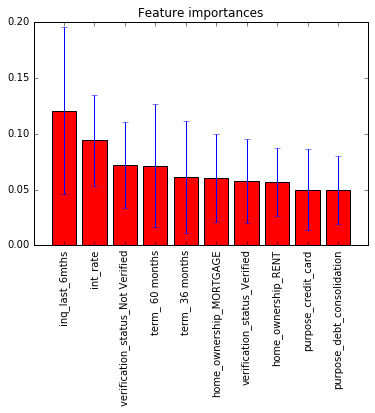### Try train and validate model on top features¶

We will select the top features based on a predetermined threshold

Extract the classifier from pipeline

In :
classifier = model_pipeline_rf.steps[-1]


Select for features based on a threshold

In :
from sklearn.feature_selection import SelectFromModel
sfm = SelectFromModel(classifier, threshold=0.01, prefit=True)

In :
X_select = sfm.transform(X)

In :
X_select = pd.DataFrame(X_select)

In :
from sklearn.cross_validation import train_test_split
X_select_train, X_select_test, y_select_train, y_select_test = train_test_split(X_select, y, test_size=0.2,
random_state=5)


Train and validate on new data set with select features to see the effect on the different algorithms

In :
classifier_name = 'Logistic Regression Classifier'
model_selectFeatures_pipeline_lr = run_cv(X_train, y_train, LogisticRegression_classifier, classifier_name)

classifier_name = 'Random Forest Classifier'
model_selectFeatures_pipeline_rf = run_cv(X_train, y_train, RandomForest_classifier, classifier_name)

classifier_name = 'Gaussian Naive Bayes'
model_selectFeatures_pipeline_nb = run_cv(X_train, y_train, GaussianNB_classifier, classifier_name)

model_selectFeatures_pipeline_gb = run_cv(X_train, y_train, GradientBoosting_classifier, classifier_name)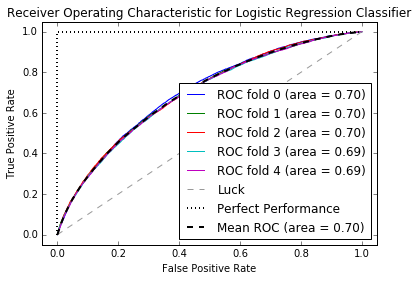Logistic Regression Classifier:
cross-validation accuracy
0.18 +/- 0.001
precision    recall  f1-score   support

0       0.97      0.01      0.02    126148
1       0.17      1.00      0.29     25884

avg / total       0.83      0.18      0.07    152032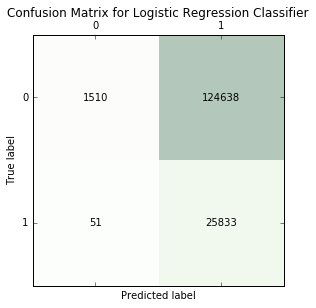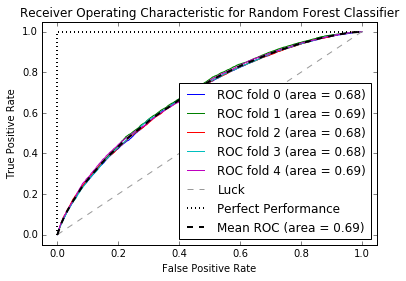Random Forest Classifier:
cross-validation accuracy
0.47 +/- 0.003
precision    recall  f1-score   support

0       0.92      0.40      0.56    126148
1       0.22      0.83      0.35     25884

avg / total       0.80      0.47      0.52    152032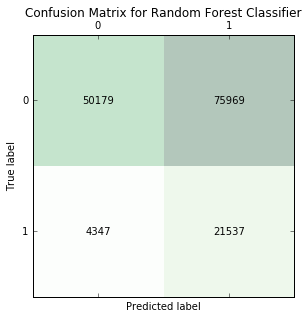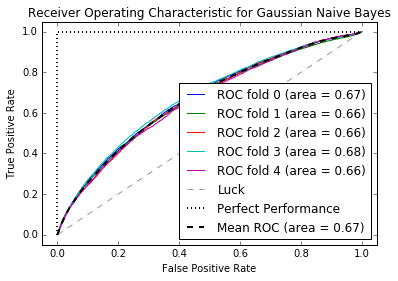Gaussian Naive Bayes:
cross-validation accuracy
0.82 +/- 0.003
precision    recall  f1-score   support

0       0.84      0.97      0.90    126148
1       0.42      0.10      0.16     25884

avg / total       0.77      0.82      0.78    152032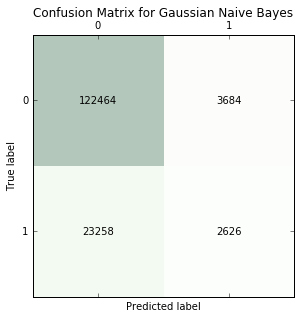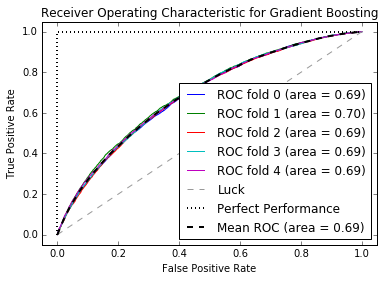Gradient Boosting:
cross-validation accuracy
0.82 +/- 0.002
precision    recall  f1-score   support

0       0.84      0.97      0.90    126148
1       0.42      0.10      0.16     25884

avg / total       0.77      0.82      0.78    152032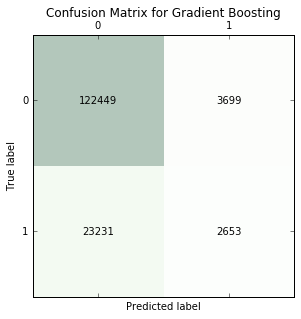### Selecting a Model¶

The random forest classifer provides the most ideal model, even with select features. We have high recall for the positive class / high precision for the negative class, meaning that we have relatively few bad loans misclassified as good. The downside is that we have very low recall for the negative class which drastically reduces the pool of loans we can invest in.

Let's now evaluate the model on the full train and test sets. We will need to make the same data transformations as the training data, and this is where using a pipeline comes in really handy.

In :
print "Random Forest Classifier"
gridsearch_rf.fit(X_select_train, y_select_train)

Random Forest Classifier

Out:
GridSearchCV(cv=2, error_score='raise',
estimator=Pipeline(steps=[('smt', SMOTE(k=5, kind='borderline1', m=10, n_jobs=-1, out_step=0.5, random_state=5,
ratio='auto')), ('scaler', StandardScaler(copy=True, with_mean=True, with_std=True)), ('classifier', RandomForestClassifier(bootstrap=True, class_weight=None, criterion='gini',
max_depth=None,...estimators=20, n_jobs=1, oob_score=False, random_state=5,
verbose=0, warm_start=False))]),
fit_params={}, iid=True, n_jobs=-1,
param_grid=[{'classifier__max_features': ['sqrt'], 'classifier__min_samples_split': , 'classifier__class_weight': [{0: 0.1, 1: 0.9}], 'classifier__n_estimators': , 'classifier__min_samples_leaf': , 'classifier__criterion': ['entropy']}],
pre_dispatch='2*n_jobs', refit=True, return_train_score=True,
scoring=None, verbose=0)
In :
gridsearch_best_estimator_rf = gridsearch_rf.best_estimator_
dill.dump(gridsearch_best_estimator_rf, open('RandomForest_gridsearch_fulltrainingset.pkl', 'wb'))

In :
print('Best parameters %s' % gridsearch_rf.best_params_)

Best parameters {'classifier__max_features': 'sqrt', 'classifier__min_samples_split': 100, 'classifier__class_weight': {0: 0.1, 1: 0.9}, 'classifier__n_estimators': 20, 'classifier__min_samples_leaf': 5, 'classifier__criterion': 'entropy'}


Evaluation metrics on the full training and test data sets

In :
print('Training accuracy:', gridsearch_rf.score(X_select_train, y_select_train))
print('Test accuracy:', gridsearch_rf.score(X_select_test, y_select_test))

('Training accuracy:', 0.54914097032203746)
('Test accuracy:', 0.49081772258471901)

In :
from sklearn.metrics import classification_report
print 'Training accuracy: %.2f' % (gridsearch_rf.score(X_select_train, y_select_train))
print('Training classification report')
print classification_report(y_select_train, gridsearch_rf.predict(X_select_train))

Training accuracy: 0.55
Training classification report
precision    recall  f1-score   support

0       0.98      0.47      0.63    126148
1       0.27      0.95      0.42     25884

avg / total       0.86      0.55      0.60    152032


In :
print 'Test accuracy: %.2f' % (gridsearch_rf.score(X_select_test, y_select_test))
print('Test classification report')
print classification_report(y_select_test, gridsearch_rf.predict(X_select_test))

Test accuracy: 0.49
Test classification report
precision    recall  f1-score   support

0       0.92      0.43      0.58     31666
1       0.22      0.80      0.35      6342

avg / total       0.80      0.49      0.54     38008



### Some results based on using different class weights¶

This is for class_weight of 0:0.10, 1:0.90

Logistic Regression Classifier: cross-validation accuracy 0.18 +/- 0.002 precision recall f1-score support 0 0.97 0.01 0.03 126148 1 0.17 1.00 0.29 25884 avg / total 0.83 0.18 0.07 152032Random Forest Classifier: cross-validation accuracy 0.48 +/- 0.003 precision recall f1-score support 0 0.92 0.40 0.56 126148 1 0.22 0.83 0.35 25884 avg / total 0.80 0.48 0.53 152032

...same but with highly correlated variables removed (>0.96)

Logistic Regression Classifier: cross-validation accuracy 0.18 +/- 0.002 precision recall f1-score support 0 0.97 0.01 0.02 126148 1 0.17 1.00 0.29 25884 avg / total 0.83 0.18 0.07 152032Random Forest Classifier: cross-validation accuracy 0.47 +/- 0.004 precision recall f1-score support 0 0.92 0.39 0.55 126148 1 0.22 0.84 0.35 25884 avg / total 0.80 0.47 0.52 152032

This is for class_weight of 0:0.90, 1:0.10

Logistic Regression Classifier: cross-validation accuracy 0.83 +/- 0.001 precision recall f1-score support 0 0.83 1.00 0.91 126148 1 0.62 0.00 0.01 25884 avg / total 0.79 0.83 0.75 152032

This is for class_weight of 0:0.95, 1:0.05

Logistic Regression Classifier: cross-validation accuracy 0.83 +/- 0.002 precision recall f1-score support 0 0.83 1.00 0.91 126148 1 1.00 0.00 0.00 25884 avg / total 0.86 0.83 0.75 152032

This is the class_weight of 0:60, 1:0.40

Logistic Regression Classifier: cross-validation accuracy 0.75 +/- 0.001 precision recall f1-score support 0 0.88 0.82 0.85 126148 1 0.33 0.44 0.38 25884 avg / total 0.78 0.75 0.77 152032

This is the class_weight of 0:0.30, 1:0.70

Logistic Regression Classifier: cross-validation accuracy 0.39 +/- 0.003 precision recall f1-score support 0 0.94 0.29 0.44 126148 1 0.21 0.91 0.34 25884 avg / total 0.81 0.39 0.42 152032

This is the class_weight of 0:0.20, 1:0.80

Logistic Regression Classifier: cross-validation accuracy 0.27 +/- 0.003 precision recall f1-score support 0 0.95 0.12 0.22 126148 1 0.19 0.97 0.31 25884 avg / total 0.82 0.27 0.23 152032 Random Forest Classifier: cross-validation accuracy 0.69 +/- 0.003 precision recall f1-score support 0 0.88 0.71 0.79 126148 1 0.28 0.55 0.37 25884 avg / total 0.78 0.69 0.72 152032

This is the class_weight of 0:0.35, 1:0.65

Logistic Regression Classifier: cross-validation accuracy 0.46 +/- 0.001 precision recall f1-score support 0 0.93 0.38 0.54 126148 1 0.22 0.85 0.35 25884 avg / total 0.81 0.46 0.51 152032 Random Forest Classifier: cross-validation accuracy 0.80 +/- 0.001 precision recall f1-score support 0 0.85 0.92 0.88 126148 1 0.37 0.24 0.29 25884 avg / total 0.77 0.80 0.78 152032

This is the class_weight of 0:0.65, 1:0.35

Logistic Regression Classifier: cross-validation accuracy 0.78 +/- 0.001 precision recall f1-score support 0 0.87 0.87 0.87 126148 1 0.36 0.34 0.35 25884 avg / total 0.78 0.78 0.78 152032 Random Forest Classifier: cross-validation accuracy 0.83 +/- 0.002 precision recall f1-score support 0 0.83 1.00 0.91 126148 1 0.51 0.01 0.02 25884 avg / total 0.78 0.83 0.76 152032

-->